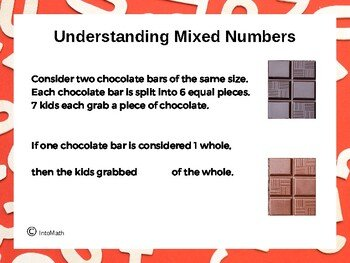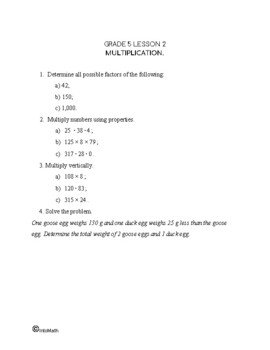CA\$2.00

We have looked at the concept of a simple fraction in one of the previous lessons.

Fractions can be proper (when the value of a number in the numerator is lower than that in the denominator) or improper (when the value of a number in the numerator is greater than or equal to that in the denominator). An improper fraction is always 1 or greater than 1.

Improper fractions can be converted into mixed numbers (also called mixed fractions) by isolating the whole in the improper fraction.

A mixed number is a combination of a whole number and a proper fraction. Rewriting an improper fraction as a mixed number can be helpful. It helps us identify more easily how many whole components there are.

Mixed numbers can be represented visually as several wholes and parts of something. For example, in the short animation below we use pizzas to demonstrate the concept. It is important to understand how to connect the visual representation of mixed numbers and their arithmetic representation on paper (tablet).

We can add and subtract mixed numbers by first adding and subtracting their whole parts and then their fractional parts. If the sum of the fractions is an improper fraction, then we change it to a mixed number. When the denominators of the fractions are different, we need to find equivalent fractions with a common denominator before adding or subtracting.CA\$4.00

#### Writing Improper Fractions as Mixed Numbers

1. Divide the denominator into the numerator

2. The quotient is the whole number of the mixed number that goes in the front

3. The remainder goes into the numerator of the fractional part of the mixed number

4. If there is another number in the numerator already, add the remainder to it

5. The denominator of the fractional part stays the same